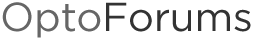# Convert IEEE 64-bit Hexal Representations to Decimal Floating-Point

This subrutine takes a String that contains the hexadecimal representation of IEEE 64 bits Double precision floating pont numbers and return the IEEE 32 bits hexadecimal representation of it and also assigns the value to a Float variable so you can work with it in your chart.

Put your IEEE 64 bits Hexa Value in the “Num_IEEE_64bits_String_Hexa” String Variable
and you could check the value in “Num_IEEE_32bits_String_Hexa” String Variable and “Num_IEEE_float” float Variable

OptoScript code:

[B]IEEE64to32(Num_IEEE_64bits_String_Hexa, Num_IEEE_float, Num_IEEE_32bits_String_Hexa);[/B]Greetings !!!